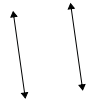# Identifying Parallel and Perpendicular Lines Online Quiz

Following quiz provides Multiple Choice Questions (MCQs) related to Identifying Parallel and Perpendicular Lines. You will have to read all the given answers and click over the correct answer. If you are not sure about the answer then you can check the answer using Show Answer button. You can use Next Quiz button to check new set of questions in the quiz.Q 1 - Use parallel or perpendicular to describe the following lines.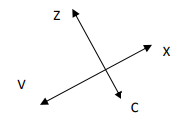### Explanation

Step 1:

Given lines are at right angles to each other.

Step 2:

So the given lines are perpendicular lines.

Q 2 - Use parallel or perpendicular to describe the following lines.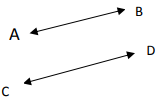### Explanation

Step 1:

Given lines are parallel to each other.

Step 2:

So the given lines are parallel lines.

Q 3 - Use parallel or perpendicular to describe the following lines.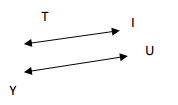### Explanation

Step 1:

Given lines are parallel to each other.

Step 2:

So the given lines are parallel lines.

Q 4 - Use parallel or perpendicular to describe the following lines.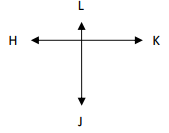### Explanation

Step 1:

Given lines are at right angles to each other.

Step 2:

So the given lines are perpendicular lines.

Q 5 - Use parallel or perpendicular to describe the following lines.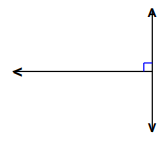### Explanation

Step 1:

Given lines are at right angles to each other.

Step 2:

So the given lines are perpendicular lines.

Q 6 - Use parallel or perpendicular to describe the following lines.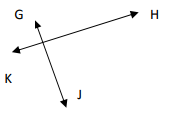### Explanation

Step 1:

Given lines are at right angles to each other.

Step 2:

So the given lines are perpendicular lines.

Q 7 - Use parallel or perpendicular to describe the following lines.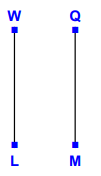### Explanation

Step 1:

Given lines are parallel to each other.

Step 2:

So the given lines are parallel lines.

Q 8 - Use parallel or perpendicular to describe the following lines.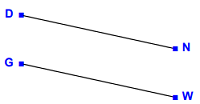### Explanation

Step 1:

Given lines are parallel to each other.

Step 2:

So the given lines are parallel lines.

Q 9 - Use parallel or perpendicular to describe the following lines.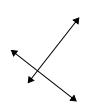### Explanation

Step 1:

Given lines are at right angles to each other.

Step 2:

So the given lines are perpendicular lines.

Q 10 - Use parallel or perpendicular to describe the following lines.